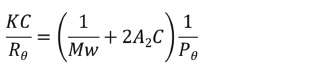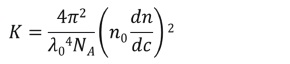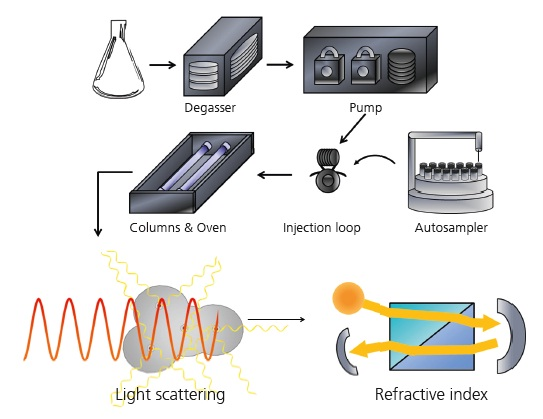# Theory of GPC/SEC Static Light Scattering

Static light scattering (SLS) employs an optical setup so that the detected signal is stable or ‘static.’ If other constants are known, then it is possible to calculate the molecular weight of a sample by measuring the intensity of light scattered by the sample. The term ‘static light scattering’ is utilized to distinguish the method from dynamic light scattering, which is a different but not unrelated technique used for particle size measurement.

## Rayleigh Theory

Rayleigh theory explains the correlation between the intensity of the light scattered by a sample and the size and molecular weight of the sample. The properties of the light scattered by particles and molecules differ based on the object scattering the light, as defined in the Rayleigh equation:Where,

• C = the sample concentration,
• θ is the measurement angle,
• Rθ = the Rayleigh ratio (the ratio of scattered light intensity to incident light intensity) at the measurement angle θ
• Mw = Weight average molecular weight,
• A2 = the second virial coeffi cient.

K and Pθ are more complex terms and are defined as follows:Where,

• λ0 is the laser wavelength in a vacuum,
• n0 is the refractive index of the solvent,
• dn/dc is the refractive index increment of the sample.Where,

• Rg is the molecule’s radius of gyration

According to the Rayleigh equation, the scattering light intensity at a given angle relies on a number of factors, which include the molecular size and molecular weight of the sample. As shown by the Rayleigh equation, the larger the molecules in size and molecular weight, the more the light will be scattered by them.

The scattering light intensity increases linearly with molecular weight, but nonlinearly with the size of the molecules. Hence, if all other factors in the Rayleigh equation are known, then the intensity of the scattered light corresponding to Rθ can be measured to calculate the molecular weight of the sample.

## Static light scattering in GPC/SEC

The SLS technique is often used for molecular weight measurements. SLS measurements can be performed in a cuvette or in a gel-permeation chromatography (GPC)/size-exclusion chromatography system (SEC). The use of a chromatography system eliminates many problems associated with the preparation and purification of the sample. Hence, it is the most common method used to implement SLS.

Moreover, GPC/SEC enables easy integration of the light scattering data with the data obtained from a concentration detector to determine the concentration of the sample simultaneously. A refractive index detector (RI) is the most commonly used concentration detector, but an ultraviolet (UV) absorbance detector can also be employed.Figure 1. Schematic of a GPC/SEC system.

The schematic of a GPC/SEC system is shown in Figure 1. The degasser, columns, injection loop and pump are standard, with the optional autosampler. The molecules are measured by one or more detectors in a given order. Here, the RI detector is positioned after the light scattering detector.

Malvern Panalytical provides the materials and biophysical characterization technology and expertise that enables scientists and engineers to investigate, understand and control the properties of dispersed systems.

These systems range from proteins and polymers in solution, particle and nanoparticle suspensions and emulsions, through to sprays and aerosols, industrial bulk powders and high concentration slurries. Used at all stages of research, development and manufacturing, Malvern Panalytical’s instruments provide critical information that helps accelerate research and product development, enhance and maintain product quality and optimize process efficiency.This information has been sourced, reviewed and adapted from materials provided by Malvern Panalytical.

## Citations

• APA

Malvern Panalytical. (2019, September 03). Theory of GPC/SEC Static Light Scattering. AZoM. Retrieved on October 26, 2020 from https://www.azom.com/article.aspx?ArticleID=9820.

• MLA

Malvern Panalytical. "Theory of GPC/SEC Static Light Scattering". AZoM. 26 October 2020. <https://www.azom.com/article.aspx?ArticleID=9820>.

• Chicago

Malvern Panalytical. "Theory of GPC/SEC Static Light Scattering". AZoM. https://www.azom.com/article.aspx?ArticleID=9820. (accessed October 26, 2020).

• Harvard

Malvern Panalytical. 2019. Theory of GPC/SEC Static Light Scattering. AZoM, viewed 26 October 2020, https://www.azom.com/article.aspx?ArticleID=9820.